# The stock price of Rene Co. is \$68

The stock price of Rene Co. is \$68. Investors require an 11.00% rate of return on similar stocks. If the company plans to pay a dividend of \$3.85 next year, what growth rate is expected for the company's stock price?

The value of the stock of the company is the present value of all its cash flows discounted at the required return of the investors.

If the dividends of the company are expected to grow at constant rate, then following formula can be used to value the stock: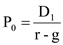Where,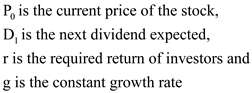.

In the given case, if the next dividend expected is \$3.85, required return is 11% and current stock price is \$68, the constant growth rate of the stock will be:Thus, the constant growth rate of the stock is 5.34%.

The value of the stock of the company is the present value of all its cash flows discounted at the required return of the investors.

If the dividends of the company are expected to grow at constant rate, then following formula can be used to value the stock:Where,.

In the given case, if the next dividend expected is \$3.85, required return is 11% and current stock price is \$68, the constant growth rate of the stock will be:Thus, the constant growth rate of the stock is 5.34%.

##### Add Answer to: The stock price of Rene Co. is \$68
Similar Homework Help Questions
• ### The stock price of Alps Co. is \$53.50. Investors require a return of 13 percent on...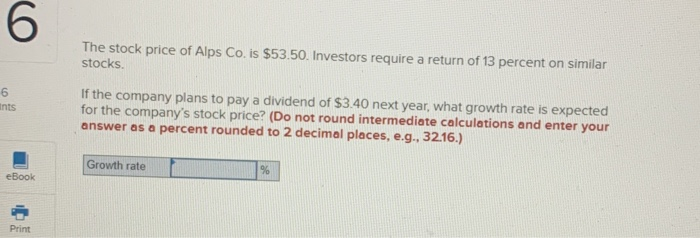The stock price of Alps Co. is \$53.50. Investors require a return of 13 percent on similar stocks. ints If the company plans to pay a dividend of \$3.40 next year, what growth rate is expected for the company's stock price? (Do not round intermediate calculations and enter your answer as a percent rounded to 2 decimal places, e.g., 32.16.) Growth rate % eBook Print

• ### Problem 7-10 Growth Rates [LO 1] The stock price of Baskett Co. is \$54.30. Investors require...

Problem 7-10 Growth Rates [LO 1] The stock price of Baskett Co. is \$54.30. Investors require a return of 13 percent on similar stocks. If the company plans to pay a dividend of \$3.80 next year, what growth rate is expected for the company’s stock price? (Do not round intermediate calculations and enter your answer as a percent rounded to 2 decimal places, e.g., 32.16.) Growth rate             %

• ### 4. A company has a stock price of \$53. Investors require a return of 12 %...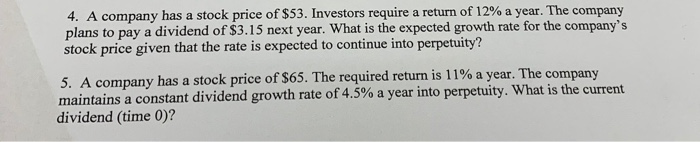4. A company has a stock price of \$53. Investors require a return of 12 % a year. The company plans to pay a dividend of \$3.15 next year. What is the expected growth rate for the company's stock price given that the rate is expected to continue into perpetuity? 5. A company has a stock price of \$65. The required return is 11% a year. The company maintains a constant dividend growth rate of 4.5 % a year into...

• ### I've noticed how there are many wrong answers to this question. Please show all steps and...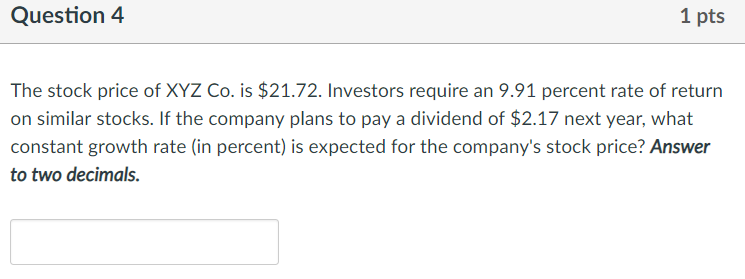I've noticed how there are many wrong answers to this question. Please show all steps and please double check your work!!! Thank you. Question4 1 pts The stock price of XYZ Co. is \$21.72. Investors require an 9.91 percent rate of return on similar stocks. If the company plans to pay a dividend of \$2.17 next year, what constant growth rate (in percent) is expected for the company's stock price? Answer to two decimals

• ### The Blooming Flower Co. has earnings of \$3.68 per share. a. If the benchmark PE for...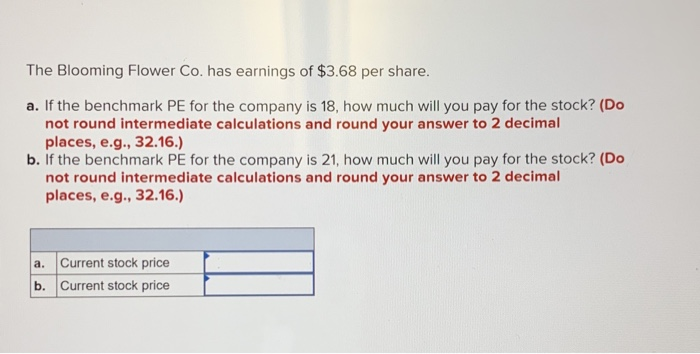The Blooming Flower Co. has earnings of \$3.68 per share. a. If the benchmark PE for the company is 18, how much will you pay for the stock? (Do not round intermediate calculations and round your answer to 2 decimal places, e.g., 32.16.) b. If the benchmark PE for the company is 21, how much will you pay for the stock? (Do not round intermediate calculations and round your answer to 2 decimal places, e.g., 32.16.) a. Current stock price...

• ### CDB stock is currently priced at \$63. The company will pay a dividend of \$4.81 next...

CDB stock is currently priced at \$63. The company will pay a dividend of \$4.81 next year and investors require a return of 11.1 percent on similar stocks. What is the dividend growth rate on this stock?

• ### CDB stock is currently priced at \$64. The company will pay a dividend of \$3.93 next...

CDB stock is currently priced at \$64. The company will pay a dividend of \$3.93 next year and investors require a return of 9.9 percent on similar stocks. What is the dividend growth rate on this stock?

• ### CDB stock is currently priced at \$78. The company will pay a dividend of \$4.49 next...

CDB stock is currently priced at \$78. The company will pay a dividend of \$4.49 next year and investors require a return of 10.7 percent on similar stocks. What is the dividend growth rate on this stock? 5.04% 5.76% 4.70% 4.94% 4.61%

• ### Dividend Growth Rate

CDB stock is currently priced at \$71. The company will pay a dividend of \$5.13 next year and investors require a return of 11.5 percent on similar stocks. What is the dividend growth rate on this stock?

• ### 3.The stock in Up-Towne Movers is selling for \$47.20 per share. Investors have a required return...

3.The stock in Up-Towne Movers is selling for \$47.20 per share. Investors have a required return of 9.8 percent and expect the dividends to grow at 4.1 percent indefinitely. What was the dividend the company just paid? 4.CDB stock is currently priced at \$72. The company will pay a dividend of \$4.25 next year and investors require a return of 10.4 percent on similar stocks. What is the dividend growth rate on this stock?

Free Homework App